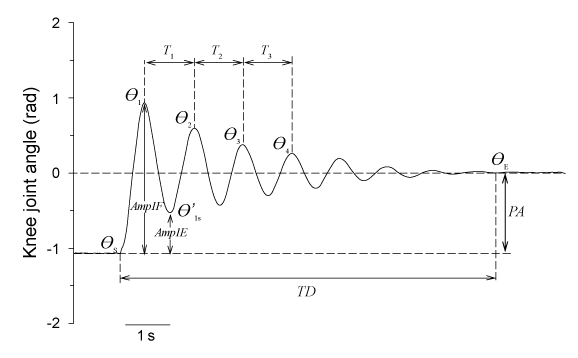Figure 1: Typical time-knee joint angle plot resulting from a leg drop pendulum test with kinematic parameters. Number of oscillations (NO: 8), angle at the start of the test (θS: -1.06 rad), angle at the end of the test (θE: 0.009rad), total duration of oscillations (TD: 8.55s), first four peak flexion angles (θ1 to θ4: 0.94, 0.60, 0.38, and 0.26rad), first peak extension angle (θ'1: -0.53rad), period of the first three cycles (T1 to T3: 1.10, 1.10, and 1.09s), plateau amplitude (PA: 1.07rad), amplitude of first flexion (Amp1F: 2rad), and amplitude of first extension (Amp1E: 0.53rad).